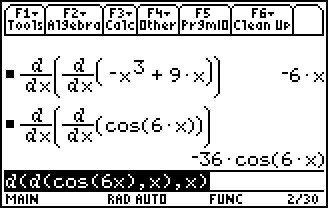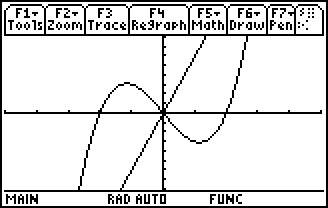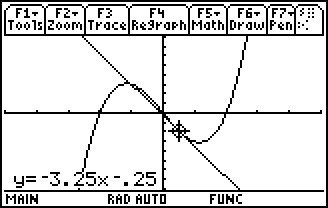# Activities

••• ##### Subject Area

• Math: Calculus: Applications of the Derivative
• Math: Calculus: Derivatives

• ##### Author9-12

45 Minutes

• ##### Device
• TI-89 / TI-89 Titanium

## Higher Order Derivatives#### Activity Overview

Students will calculate the second derivative of functions, inspect a graph and give the intervals for concave up and concave down and find the point of inflection.

#### Key Steps

•Students will explore the higher derivatives of several functions and relate these to the shape of the function graph.

Students will use a nested derivative command to find the second derivative of each function.

•Students will graph the function and its second derivative on the same screen to discover how the function's second derivative relates to the function's shape.

•Students will then find the equation of the tangent line at specific x-values. Students will use this information to find the concavity and point(s) of inflection of the function.